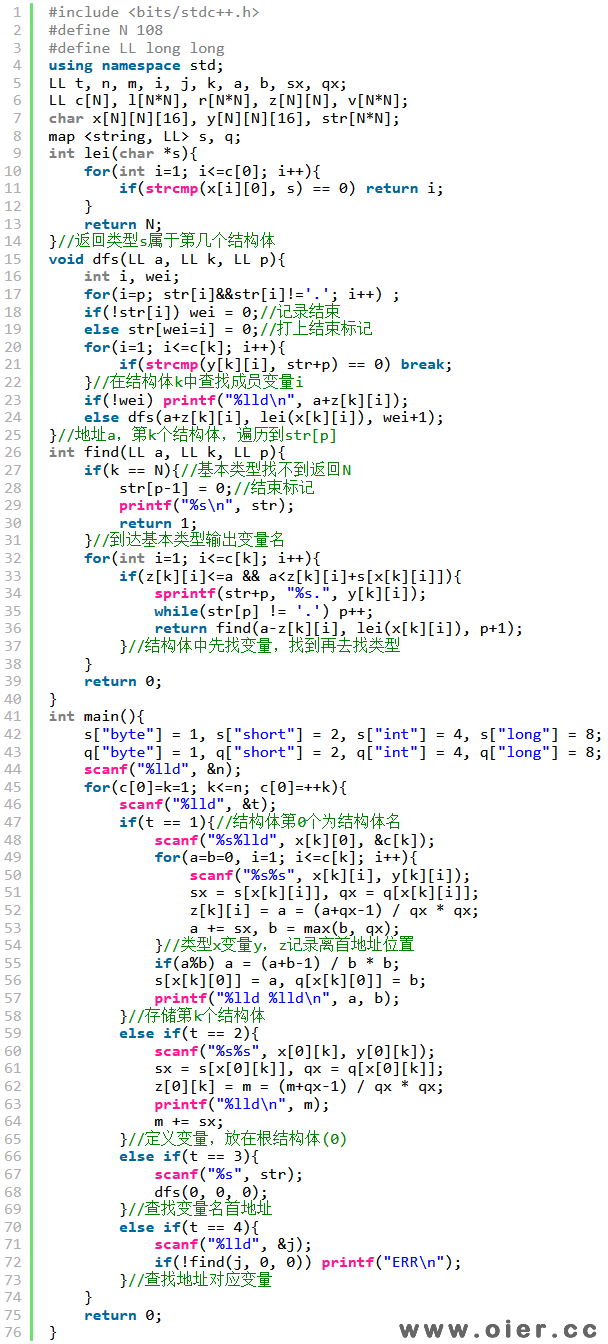78+

## 题目描述

– 元素内的所有成员将按照**定义时给出的顺序**在内存中排布，对于类型为结构体的成员同理。
– 为了保证内存访问的效率，元素的地址占用需要满足**对齐规则**，即任何类型的**大小**和该类型元素在内存中的**起始地址**均应对齐到该类型对齐要求的**整数倍**。具体而言：
– 对于基本类型：对齐要求等于其占据空间大小，如 int 类型需要对齐到 $4$ 字节，其余同理。
– 对于结构体类型：对齐要求等于其成员的对齐要求的**最大值**，如一个含有 int 和 short 的结构体类型需要对齐到 $4$ 字节。

“cpp
struct d {
short a;
int b;
short c;
};
d e;
“

1. 定义一个结构体类型。具体而言，给定正整数 $k$ 与字符串 $s, t_1, n_1, \dots, t_k, n_k$，其中 $k$ 表示该类型的成员数量，$s$ 表示该类型的类型名，$t_1, t_2, \dots, t_k$ 按顺序分别表示每个成员的类型，$n_1, n_2, \dots, n_k$ 按顺序分别表示每个成员的名称。你需要输出该结构体类型的大小和对齐要求，用一个空格分隔。

2. 定义一个元素，具体而言，给定字符串 $t, n$ 分别表示该元素的类型与名称。所有被定义的元素将按顺序，从内存地址为 $0$ 开始依次排开，并需要满足地址对齐规则。你需要输出新定义的元素的起始地址。

3. 访问某个元素。具体而言，给定字符串 $s$，表示所访问的元素。与 C++ 等语言相同，采用 . 来访问结构体类型的成员。如 a.b.c，表示 a 是一个已定义的元素，它是一个结构体类型，有一个名称为 b 的成员，它也是一个结构体类型，有一个名称为 c 的成员。你需要输出如上被访问的**最内层**元素的起始地址。

4. 访问某个内存地址。具体而言，给定非负整数 $addr$，表示所访问的地址，你需要判断是否存在一个**基本类型**的元素占据了该地址。若是，则按操作 3 中的访问元素格式输出该元素；否则输出 ERR。

## 输入输出格式

### 输入格式

– 若 $op = 1$，首先输入一个字符串 $s$ 与一个正整数 $k$，表示类型名与成员数量，接下来 $k$ 行每行输入两个字符串 $t_i, n_i$，依次表示每个成员的类型与名称。

– 若 $op = 2$，输入两个字符串 $t, n$，表示该元素的类型与名称。

– 若 $op = 3$，输入一个字符串 $s$，表示所访问的元素。

– 若 $op = 4$，输入一个非负整数 $addr$，表示所访问的地址。

## 输入输出样例

### 输入样例 #1

5
1 a 2
short aa
int ab
1 b 2
a ba
long bb
2 b x
3 x.ba.ab
4 10

### 输出样例 #1

8 4
16 8
0
4
x.bb

## 说明

#### 【样例 1 解释】

#### 【样例 2】

#### 【样例 2 解释】

#### 【样例 3】

#### 【数据范围】

| 测试点 | 特殊性质 |
| :———-: | :———-: |
| $1$ | A、D |
| $2\sim 3$ | A |
| $4\sim 5$ | B、D |
| $6\sim 8$ | B |
| $9\sim 10$ | C、D|
| $11\sim 13$ | C|
| $14\sim 16$ |D|
|$17\sim 20$| 无|

#### 【提示】

– 设该结构体内有 $k$ 个成员，其大小分别为 $s_1,…,s_k$，对齐要求分别为 $a_1,…,a_k$;
– 则该结构体的对齐要求为 $a=\max\{a_1,…,a_k\}$；
– 再设这些成员排布时的**地址偏移量**分别为 $o_1,…,o_k$，则：
– $o_1 = 0$;
– 对于 $i=2,…,k$，$o_i$ 为满足 $o_{i-1}+s_{i-1}\le o_i$ 且 $a_i$ 整除 $o_i$ 的最小值；
– 则该结构体的大小 $s$ 为满足 $o_k+s_k\le s$ 且 $a$ 整除 $s$ 的最小值；

– 设第 $i$ 个被定义的元素大小为 $s_i$，对齐要求为 $a_i$，起始地址为 $b_i$;
– 则 $b_1 = 0$，对于 $2\le i$， $b_i$ 为满足 $b_{i-1} + s_{i-1}\le b_i$ 且 $a_i$ 整除 $b_i$ 的最小值。

## 解题思路

15分：没有操作1，记录每个变量的首地址，记录每个地址属于哪个变量即可；

65分：将所有变量、结构体、结构体.成员的全称及其地址范围存储下来即可，因为不存在结构体嵌套，所以是不会超时的。需要注意的是，输出的是基本类型的变量，结构体本身不要存储范围，只需要存储首地址。

100分：定义的变量，看成是第0个结构体的成员变量；定义的结构体，依次保存到第k个结构体，包括成员变量的类型x和变量名y，以及该变量里结构体首地址的距离z、成员数量c。对于变量名查找地址，从0号结构体开始，逐个变量名查找：在结构体中遍历成员变量，找到相同的变量名，再去其类型继续找下一级变量，直到找完整个变量名。对于地址找变量，从0号结构体开始，依次遍历结构体里的每个成员，只要成员地址范围包含该地址，就进去该成员的结构体继续找，如果在空隙找不到，就输出ERR。

## 程序实现